PH316 Non-Linear Phenomena and Chaos.

### Summer 1998

1.

What is the mathematical distinction between linear and nonlinear operators? Define linear deficiency and provide an example.

Describe the importance of the role of dissipation in nonlinear dynamics and include some examples from physical systems.

Define quasi-periodic motion and describe the various means by which chaotic motion may be distinguished from that which is quasi-periodic.

Comment on the differences between mappings and flows in terms of when they may or may not exhibit chaotic behaviour.

Discuss either how a chaotic system may be controlled or the synchronization of chaotic systems.

2.

For 1D flows explain the meaning of the terms attractor and repellor.

Write explanatory notes on the following types of bifurcations which occur in 1D systems: (i) saddle-node, (ii) transcritical, (iii) supercritical pitchfork, (iv) subcritical pitchfork.
In each case give the normal form of the equation of motion that characterises the type of bifurcation and the phase-plane behaviour as the control parameter passes through the critical point. Include a brief description of one physical example of a bifurcation.

Use linear stability analysis to study the behaviour of the 1D system: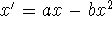when a and b are positive constants.

Give sketches of appropriate phase-plane plots to illustrate your results.
Include sketches of the phase-plane behaviour and potentials when a and b can take negative values.

What happens to the fixed points as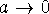?

3.

Write down the general equations for the case of 2D autonomous flows, then define what is meant by: (i) elliptic points,
(ii) hyperbolic points, (iii) stable and unstable spirals, (iv) stable and unstable nodes.

Write the following nonlinear second order differential equation: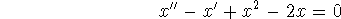as a pair of coupled first order differential equations.
Find all of the fixed points and use linearisation to classify them.

Sketch the global behaviour of the phase portrait.

4.

Describe which types of attractors arise in:
(i) 2D nonlinear systems of ordinary differential equations,
(ii) 3D nonlinear systems of ordinary differential equations.

Consider the equation: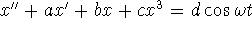where a, b, c, d and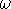are constants.

Describe carefully in geometric terms the dynamic properties of the solutions for the case a = 0, d = 0 but with b and c taking non-zero values.

Discuss effects on the dynamics first of letting a become non-zero then allowing d (and) to become non-zero.

Sketch typical Poincaré plots you might observe with this system paying particular attention to the effects of the changing magnitude of a.

5.

Explain what is meant by the orbit of x under a 1D mapping f in real space.
What are the fixed points of f?
Define the periodic points of f.
Define the Lyapunov exponents of f.
What is an invertible map?
Why is it of interest to know whether a map is invertible or non-invertible?

Consider the family of maps defined by the function:
f(x) = r x if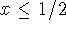r (1 - x) if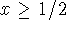where r is positive.
Show that for r < 1 the only fixed point is an attractor at the origin.
Show that for r > 1 there are two unstable fixed points.
Show that for r = 2 these two repellors are at x = 0 and x = 2/3.
Determine the Lyapunov exponent.

Indicate how the dynamical properties of the slope-3 (r=3) map differ from those of the slope-2 (r=2) map.

6.

Write concise explanatory notes on THREE of the following:

(a) the use of nullclines to determine global behaviour of the phase portrait of a system such as:x' = xy -y, y'= yx -x ,

(b) Hopf bifurcations and their occurrence in physical systems,

(c) the definition and use of the various dimensions of fractal type,

(d) the use of the Kaplan-Yorke conjecture,

(e) the various routes to chaos.

END

Mike Wilson

Mon Aug 3 16:29:32 BST 1998